Biomedical Sciences
Volume 3, Issue 1, January 2017, Pages: 1-5

Noninvasive Method Calculating of Volume of Cavities of the Heart According to the Echocardiography

Faxriddin Abdikarimov Baxromovich, Kuralbay Navruzov Navruzovich

Department of Applied Mathematics, Physics and Mathematics Faculty, Urgench State University, Urgench, Uzbekistan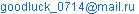(F. A. Baxromovich)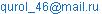(K. N. Navruzovich)

Faxriddin Abdikarimov Baxromovich, Kuralbay Navruzov Navruzovich. Noninvasive Method Calculating of Volume of Cavities of the Heart According to the Echocardiography. Biomedical Sciences. Vol. 3, No. 1, 2017, pp. 1-5. doi: 10.11648/j.bs.20170301.11

Received: November 29, 2016; Accepted: January 14, 2017; Published: February 9, 2017

Abstract: The article reveals the determination of the volume of the cavities of the heart ventricles by echocardiography, which is important in cardiology. Paper focuses on the left ventricle of the heart, which has the most correct and most accessible form of visualization. Measuring the ultrasonic probe by setting it in the parasternal short-axis positions at the end of the mitral valve determines the area of the cavity length and by. Then, the ultrasonic sensor is installed in the apical four-access. The image is recorded, investigated the cavity of the long axis is split evenly n segments. Parallel lines held perpendicular to the interventricular septum at each point of division. Each short axis corresponds to the definition area of the cavities of the ventricles, which are also perpendicular to the interventricular septum. Corresponding to each segment of the ventricular cavity volume is determined by calculating the approximate cubature formula for Simpson. Then, summing up all the calculated amounts, we finally obtain the formula for determining the volume of the cavity of the ventricles of the heart. It states that this method is more accurate and reliable than other existing methods.

Keywords: Echocardiography, Ultrasound, Mitral, Apical, Non-invasive, Cubature

Contents

1. Introduction

Problems in determining the volumetric parameters by echocardiography, essentially similar to the problems are associated with the restoration of the full form of the heart. The researchers always hampered by difficulty the visualization parts of the heart, and above all the ventricles, arising from the specific features carrying out ultrasonic research. Therefore, echocardiography is no single approach to the calculation of volume indicators heart cavities. The large number of proposed in the literature approaches to the assessment of volume indicators of the heart cavities, based on the one and two-dimensional images [1, 2, 3, 4, 5]. The results suggest that the problem of choosing the optimal ways of calculating volume indicators is not completely finished. However, the blood circulation parameters of the cardiovascular system is calculated based on a more accurate measurement of the volume of ventricular cavities, and its value can be biased to influence the final overall diagnosis. In this connection, a more accurate determination of the volume of ventricular cavities, non-invasive method has important value at treatment and prognosis of cardiovascular diseases. The variety methods of calculating the volume of the ventricular chambers are caused by the complexity of their spatial configuration. This makes it necessary submission of cavities spatial figure of regular geometric shape. Such model allows calculating the volume of the cavity indicators, having only a few of its linear sizes. Basically researchers focused attention on the left ventricle as having the most proper shape and the most well available imaging. The most common way of calculating volume of the left ventricle is a calculation formula Teychholtsa (Teichholz) [3, 5], where the volume of the ventricular cavity calculated approximately spherical volume. There are others methods of calculation , based on the ellipsoid model and also on the basis of representation of the left ventricle in the form of an ellipsoid of revolution. All these calculations lead eventually to the calculation of the volume of spherical cavities volumes. There is also not taken into account the size of the long axis, which is essential for a more accurate calculation of the volume of the cavity. Depending on the available pathology [3, 5] to describe the shape of the left ventricle, also apply hyperboloid, a hemisphere, a truncated sphere and the other rotation figure. The major disadvantages of these works [2, 3, 5], too, are an adequate reflection of the configuration of the normal and the pathological state of the human heart, the lack of comparability of the results of calculating the volume of a cavity different methods and, as a consequence, the lack of uniform criteria for pathology. The most essential to determine the volume of the left ventricle, can be considered a method of cross sections. The division of volume into n similar volumes, the work done in , where the shape of internal and external contours of ventricular short axis, despite the complex character, is preserved at the cut of these areas along the long axis of the heart. The presence of this singularity confirms the statistical analysis of data obtained as a result postmortem studies serial sections of human heart. Using these features,  the ventricular volume of the heart is calculated, having only two sections: on the short and long axes in terms of echocardiography - parasternal short axis at the end of the mitral valve and apical four-chambered access. The volume of the left ventricular cavity eventually reduces to the calculation of the cylindrical volume. Therefore, the accuracy of the formula , is low. In this paper, the aim is to propose methods that determine a more accurate measurement of the heart ventricle, through the use of echocardiography data.

2. Method

Measurements of volume ventricular cavities, in a certain sense depend on the correct scan of the heart ventricles by ultrasonic devices. Below are some methods to scan the heart chambers. As is known, the location of the left and right lung of the sternum, of ribs and narrow intercostal spaces complicates echocardiographic study. In order to facilitate access to the heart of ultrasonic methods of research, there are a number of ways, for example, scanning should be done in the patient's position on the left side, with his back to the researcher or by the person to the researcher, etc. In this regard, in echocardiography is using a number of approaches to the heart, such as a left parasternal, apical, subcostal, suprasternal, parasternal right, and right and left supraclavicular (Figure 1. and Figure 2.).1-left parasternal access, 2-apical access, 3-subcostal access, 4-suprasternal access, 5-right parasternal access, 6-right and left supraclavicular approaches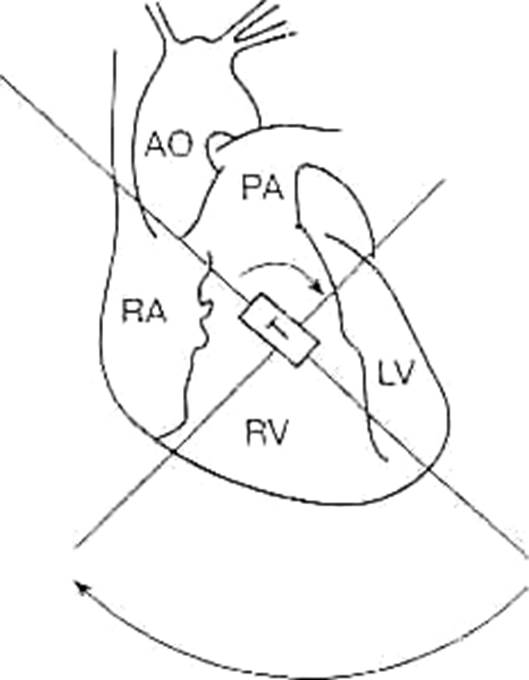Figure 2. The scheme of orthogonal projections at carrying out echocardiographic study .

In each of these approaches, depending on the position sensor can be obtained on the long the slice of the heart or the short axis. The screen shows, or mirror inverted the actual picture. The mirror image implies removing the left chambers of the heart to the right to the screen. Basically, here we restrict the two scanning positions, which is important for determining the volume the ventricles of the heart. The first position is the parasternal short axis. For this position sensor you must install at the end of the mitral valve. The screen shows the slice of the left ventricular short-axis, and the top left of the screen is the right ventricle in the form of a crescent is attached to the left ventricle  (Figure 3.).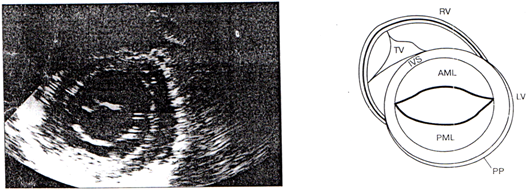Figure 3. Short-axis parasternal position on at the end of the mitral valve.

The position of the heart in the thorax may be different, and therefore the right ventricle can be located on the screen at different people differently. It is connected with this small informative value of the right ventricle size measurement in parasternal position. Order to get the necessary data to measure the volume of the left ventricle of the heart, it is necessary to install the sensor at the level of the mitral valve, and then, you need to gently move the sensor to the side of the apical impulse. Each time the marked points are measured long and short cut-off diameters. In the second case, the measurement made on the apical four-chamber position. Sensor is installed in the region of the apical impulse and direction beam is conditionally into the patient's right shoulder from the bottom up, as far as possible is necessary to obtain an image of the heart along the long axis  (Figure 4).Figure 4. The apical four-chamber position (not shown in the atrium).

The traditionally accepted echocardiography works with a mirror image on the screen so the left ventricle and the left atrium located on the right and the right ventricle and the right atrium to the left. This position is well visible mount the left and right fibrous rings at different levels right shifted into the right ventricle of 5-7 mm from the left. Using data obtained by echocardiography, calculated volume of the cavity of the left ventricle by various methods: methods of separating the volume of cavities on the cylindrical volume, methods of separating the cavity volume of a truncated cone and methods of separating the volume of curved cylindrical volumes. Below we consider the method of dividing the volume of the cavities on the n- curved cylindrical volume, where the volume of cavity measurements is made exactly to any known (cylindrical, conical, intersecting conical, cylindrical cam, and etc) geometric shapes.

3. Discussion

In contrast to the above work, there are defined first area and length of the cavity in the parasternal position sensor for installing the short axis at the end of the mitral valve (Figure 5.). Next, the ultrasonic sensor is installed in the apical four-chamber access. The image fixed by, the studied the cavity along the long axis is divided evenly into n segment (Figure 6.), as is done in [7, 8, 9, 10]. For each point of its division is conducted parallel to a line perpendicular to the interventricular septum (Figure 5.).Figure 5. Equal septum of the long axis of the n-segments, each segment containing the curved volume of a truncated cone.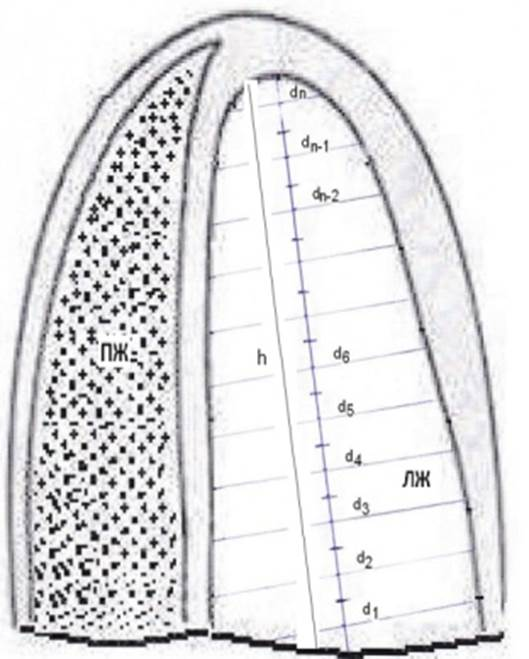Figure 6. The apical four-chamber position (not shown in the atrium).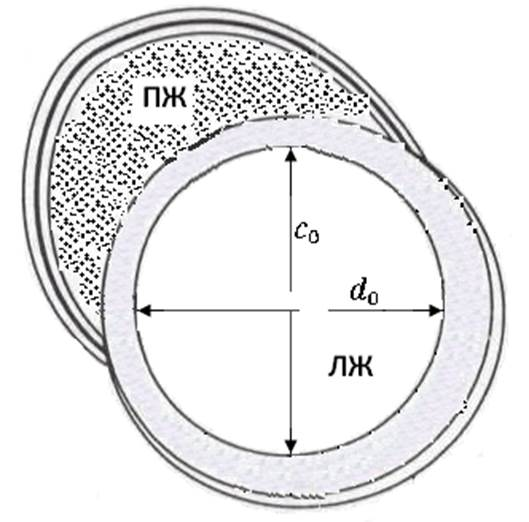Figure 7. Parasternal position on the short axis at the level of the end flaps of the mitral valve.

Each short axis corresponds to certain areas, which is also perpendicular to the interventricular septum (Figure 7.). Similar areas are determined by using the formula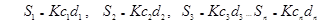(1)

where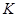proportionality coefficient,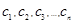- parallel axes,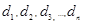- perpendicular axes to an interventricular septum. From condition of similarity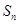of plane figures, it follows that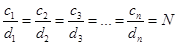(2)

From here is easy to find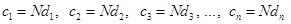(3)

Using (3) to (1) we define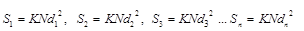(4)

Now, lump volume ventricular cavities define by calculating the approximate volume of truncated cone of curvilinear cubature formula Simpson. In this case, each segment corresponds to certain volume curvilinear truncated cone, which is calculated by the formula(5)

Considering the similarity area in each heart section long axis, and given in (5) in the form of their values (4), we obtain the following formula for determining the ventricular volumes lumpy cavities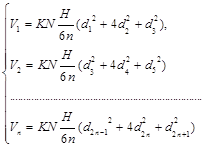(6)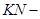the coefficient is defined from any fixed the areas of similar plane figures . Therefore we denote it fixed.(7)

4. Result

Substituting (7) into (5), and summing the volumes of all, we finally find the formula for calculating the volume of ventricular cavities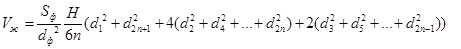(8)

where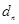– the size along the short axis, held at the point of division n-th segment perpendicular to the interventricular septum.– the size of the long axis of the ventricle;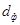– fixing the size of the cavity in the access parasternal short axis;– fixation in the area of the cavity access parasternal short axis;– dimensionless geometric factor of similar plane figures.

5. Conclusion

Our proposed method can achieve the following effect: a sharp reduction in analysis time echocardiographic survey data, establish a more accurate measurement of ventricular cavity and reliable to put the diagnosis to the patient, when calculating the volume known geometric shapes (sphere, cone, ellipsoid, etc.), This method allows that it determines its exact volume found in formula (8) to calculate the amount of ventricular cavity mathematically justified, and each member has a physical meaning.

References

1. Tolyzhnikov V. A., Semenova E. N. Basic principles of calculation of volume indicators of heart//Cardiology, 1987. t.27. 6, S. 119-123.
2. Alpatov A. V. Way of scoping of cavities of ventricles of heart//№ 2194450, 2002.
3. Teichholz L. E., Kreulen T., Herman M. V., Gorlin R. Problems in echocardiographic volume determinations: echocardiographic-angiographic correlations in presence or absence of asynergy // Amer. J. Cardiology. – 1976. – Vol. 3. – P. 7.
4. Mukharlyamov N. M., Belenkov Y. N. Ultrasonic diagnostics in cardiology - M:, "Medicine", 1981, 157 pages.
5. Clinical ultrasonic diagnostics//Under the editorship of Mukharlyamov. - М: 1987, t.1.185 pages.
6. Clinical guide to ultrasonic diagnostics//Pod. Red of Mit'kov V. V., Sandrikov V. A. - М: Vidra, 1998, 350 pages.
7. Abdikarimov F. B., Navruzov Q. N., Khujatov N. J. New way scoping of a cavity of the left ventricle of heart according to an echocardiography //European Science review, Vienna, №2 2014 (March-Aprel), p.44-46.
8. Abdikarimov F. B., Navruzov Q. N.,Khujatov N. J., Babajanova Y. I.Ultrasonic methods determining volume of cavities of ventricles of heart.//European Journal of Biomedical and Life Sciences, Vienna, 2014.№ 2.(March-Aprel).
9. Abdikarimov F. B., Navruzov Q. N., Khujatov N. J., Babajanova Y. I.Themethodofcalculatingthevolumeofthecavitiesoftheheartventricles.//The USA Journal of Applied Sciences, Nyu York, 2015.№ 2.(March-Aprel).
10. Abdikarimov F. B., Navruzov Q. N., Khujatov N. J. New way scoping of a cavity of the left ventricle of heart according to an echocardiography. //International journal of applied and fundamental research №8, 2014, Moscow.

 Contents 1. 2. 3. 4. 5.
Article ToolsAbstractPDF(1227K)